Courses

# Worksheet Question - Symmetry Class 6 Notes | EduRev

## Class 6 : Worksheet Question - Symmetry Class 6 Notes | EduRev

The document Worksheet Question - Symmetry Class 6 Notes | EduRev is a part of the Class 6 Course Mathematics (Maths) Class 6.
All you need of Class 6 at this link: Class 6

Q.1. In the following figures, l is the line of symmetry. Complete the diagram to make it symmetric.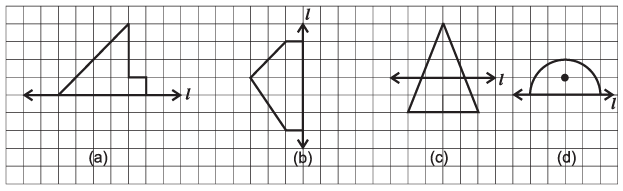Ans.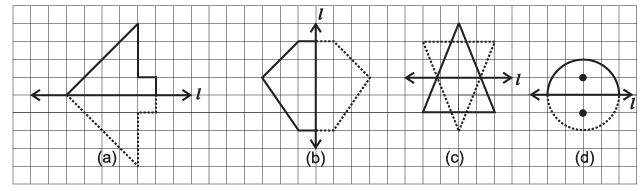Q.2. Draw the line (s) of symmetry for each of the following figures :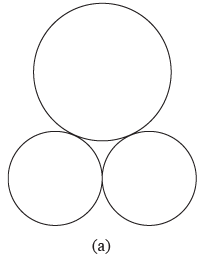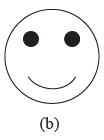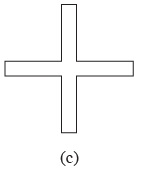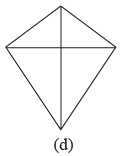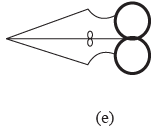Ans.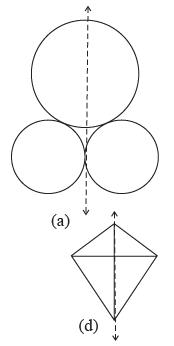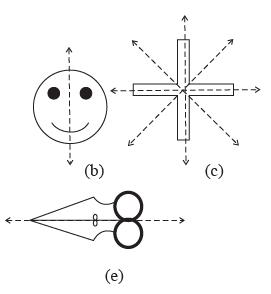Q.3. Match the following :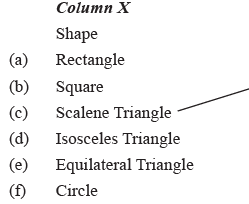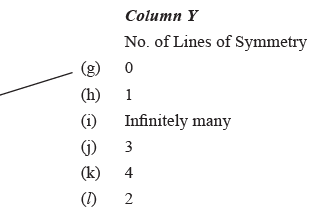For example : A scalene triangle has no line of symmetry, so (c) is matched with (g).

Ans.
(a) ↔ (l)
(b) ↔ (k)
(c) ↔ (g)
(d) ↔ (h)
(e) ↔ (j)
(f) ↔ (i)

Q.4. Consider the first ten capital letters of English alphabet, list among them the letters which have :
(i) Vertical lines of symmetry (e.g. A)
(ii) Horizontal lines of symmetry (e.g. B)
(iii) No lines of symmetry (e.g. F)
(iv) Both-vertical and horizontal lines of symmetry (e.g. H)

Ans.
(i) A, H, I
(ii) B, C, D, E, H, I
(iii) F, G, J
(iv) H, I

Q.5. State whether the following statements are True or False :
(i) Perpendicular bisector of a line segment is also its line of symmetry.
(ii) Angle bisector of an angle, with equal arms, is also its line of symmetry.
(iii) All the chords of a circle are its lines of symmetry.
(iv) A pentagon can have exactly one line of symmetry.
(v) A regular octagon has 8 lines of symmetry.
(vi) Two diagonals of a rectangle are its lines of symmetry.

(vii)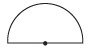This figure has 4 lines of symmetry.

Ans.
(i) True
(ii) True
(iii) False
(iv) False
(v) True
(vi) False
(vii) False

Offer running on EduRev: Apply code STAYHOME200 to get INR 200 off on our premium plan EduRev Infinity!

## Mathematics (Maths) Class 6

191 videos|220 docs|43 tests

,

,

,

,

,

,

,

,

,

,

,

,

,

,

,

,

,

,

,

,

,

;